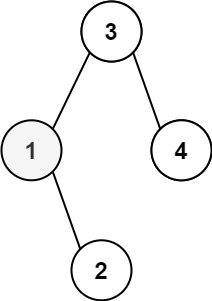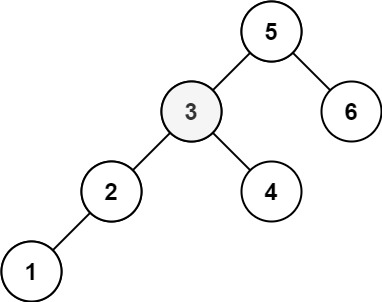• 树中的节点数为 n 。
• 1 <= k <= n <= 104
• 0 <= Node.val <= 104

## 解题代码

]]>

「外观数列」是一个整数序列，从数字 1 开始，序列中的每一项都是对前一项的描述。

• countAndSay(1) = “1”
• countAndSay(n) 是对 countAndSay(n-1) 的描述，然后转换成另一个数字字符串。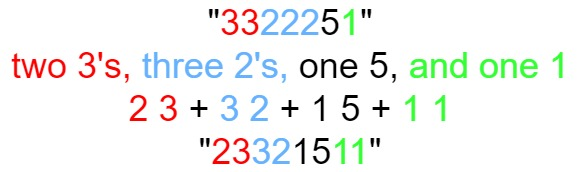• 1 <= n <= 30

## 解题代码

]]>
<h2 id="题目：38-外观数列"><a href="#题目：38-外观数列" class="headerlink" title="题目：38. 外观数列"></a>题目：38. 外观数列</h2><p>难度：中等</p> <p>给定一个正整数 n ，输出外观数列的第 n 项

• arr.length >= 3
• 存在 i（0 < i < arr.length - 1）使得：
• arr < arr < ... arr[i-1] < arr[i]
• arr[i] > arr[i+1] > ... > arr[arr.length - 1]

• 3 <= arr.length <= 104
• 0 <= arr[i] <= 106
• 题目数据保证 arr 是一个山脉数组

## 解题代码

]]>

• answer[i] == “FizzBuzz” 如果 i 同时是 3 和 5 的倍数。
• answer[i] == “Fizz” 如果 i 是 3 的倍数。
• answer[i] == “Buzz” 如果 i 是 5 的倍数。

• 1 <= n <= 104

## 解题代码

]]>

• 被除数和除数均为 32 位有符号整数。
• 除数不为 0。
• 假设我们的环境只能存储 32 位有符号整数，其数值范围是 [−231, 231 − 1]。本题中，如果除法结果溢出，则返回 231 − 1。

## 解题代码

]]>

• 0 <= num <= 231 - 1

## 解题代码

]]>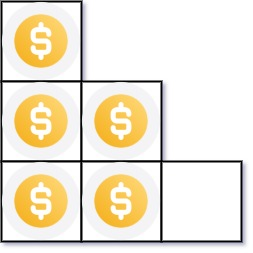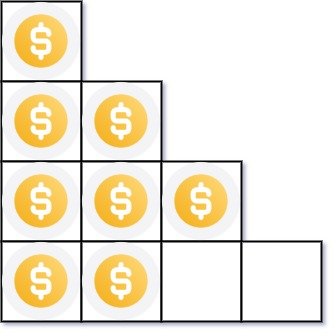• 1 <= n <= 231 - 1

## 解题代码

]]>

• 0 <= s.length <= 105
• s[i] 为 ‘A’、’C’、’G’ 或 ‘T’

]]>

## 解题代码

]]>

• 1 <= nums.length <= 104
• -231 <= nums[i] <= 231 - 1

## 解题代码

]]>

• PeekingIterator(int[] nums) 使用指定整数数组 nums 初始化迭代器。
• int next() 返回数组中的下一个元素，并将指针移动到下个元素处。
• bool hasNext() 如果数组中存在下一个元素，返回 true ；否则，返回 false 。
• int peek() 返回数组中的下一个元素，但 移动指针。

• 1 <= nums.length <= 1000
• 1 <= nums[i] <= 1000
• 对 next 和 peek 的调用均有效
• next、hasNext 和 peek 最多调用 1000 次

## 解题代码

]]>

1. S 的长度可能很长，请按需分配大小。K 为正整数。
2. S 只包含字母数字（a-z，A-Z，0-9）以及破折号’-‘
3. S 非空

## 解题代码

]]>

1. 十六进制中所有字母(a-f)都必须是小写。
2. 十六进制字符串中不能包含多余的前导零。如果要转化的数为0，那么以单个字符’0’来表示；对于其他情况，十六进制字符串中的第一个字符将不会是0字符。
3. 给定的数确保在32位有符号整数范围内。
4. 不能使用任何由库提供的将数字直接转换或格式化为十六进制的方法。

## 解题代码

]]>

• 1 <= paths.length <= 100
• paths[i].length == 2
• 1 <= cityAi.length, cityBi.length <= 10
• cityAi != cityBi
• 所有字符串均由大小写英文字母和空格字符组成。

## 解题代码

]]>

• 第一个矩形由其左下顶点 (ax1, ay1) 和右上顶点 (ax2, ay2) 定义。
• 第二个矩形由其左下顶点 (bx1, by1) 和右上顶点 (bx2, by2) 定义。• -104 <= ax1, ay1, ax2, ay2, bx1, by1, bx2, by2 <= 104

## 解题代码

]]>

• n == machines.length
• 1 <= n <= 104
• 0 <= machines[i] <= 105

## 解题代码

]]>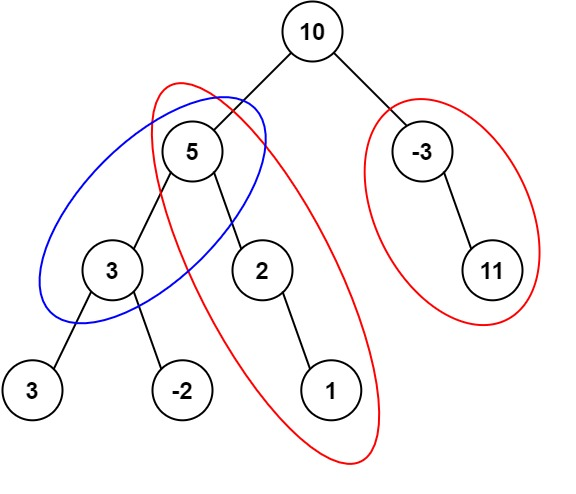• 二叉树的节点个数的范围是 [0,1000]
• -109 <= Node.val <= 109
• -1000 <= targetSum <= 1000

## 解题代码

]]>

• -1000 <= a, b <= 1000

## 解题代码

]]>
<h2 id="题目：371-两整数之和"><a href="#题目：371-两整数之和" class="headerlink" title="题目：371. 两整数之和"></a>题目：371. 两整数之和</h2><p>难度：中等</p> <p>给你两个整数 a 和 b ，<

1. 给定单词的长度不超过500。
2. 给定单词中的字符只含有小写字母。

## 解题代码

]]>• 节点数目不超过 1000
• 1 <= Node.val <= 10^5

]]>